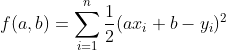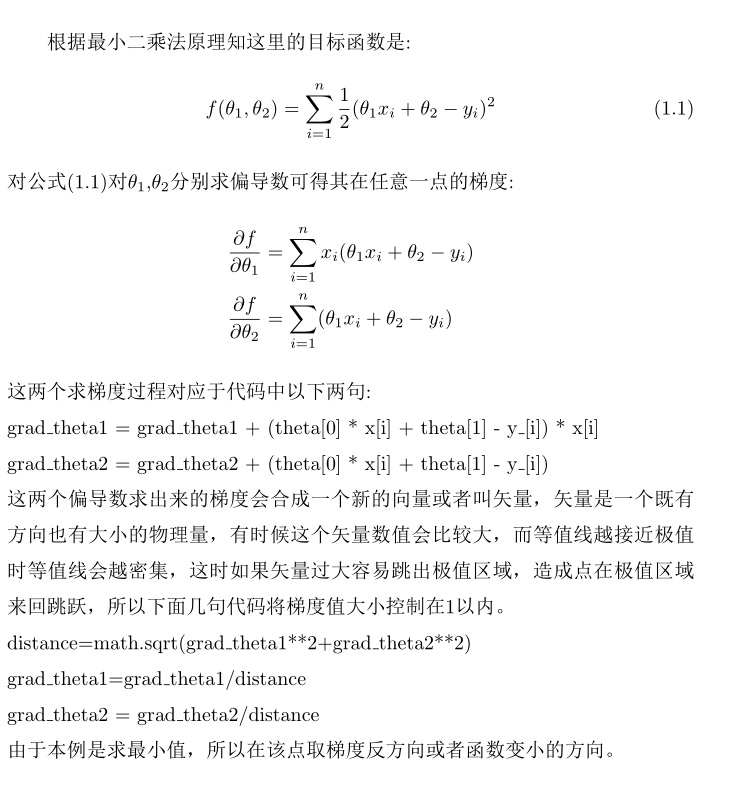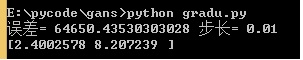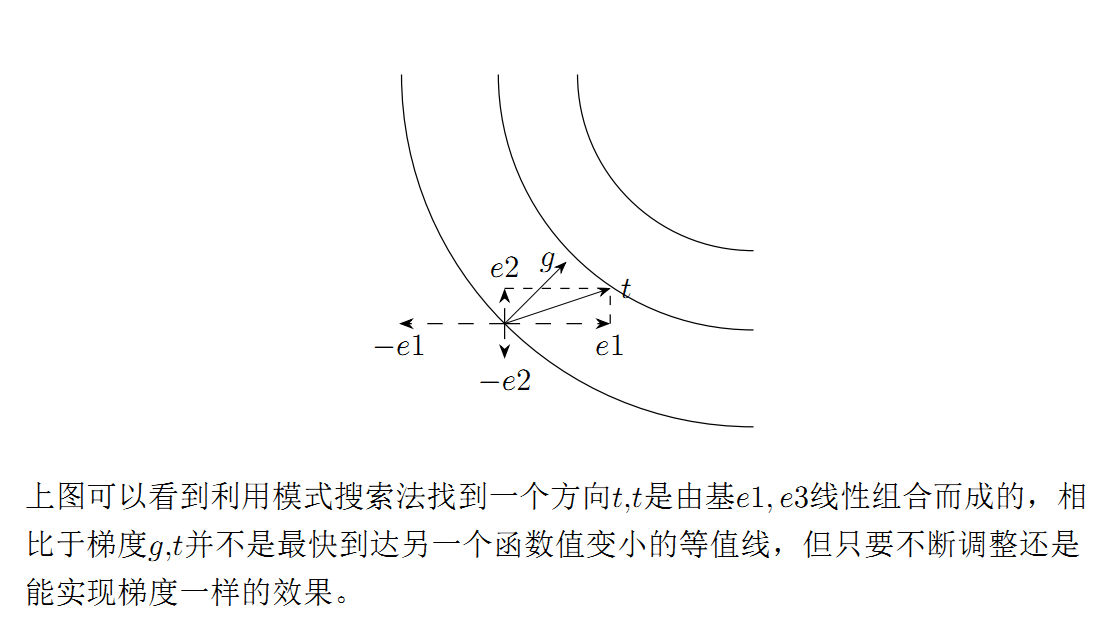梯度法、模式搜索法求解最优化问题

最优化问题中常常需要求解目标函数的最大值或最小值，比如SVM支持向量机算法需要求解分类之间最短距离，神经网络中需要计算损失函数的最小值，分类树问题需要计算熵的最小或最大值等等。如果目标函数可求导常用梯度法，不能求导时一般选用模式搜索

由数学分析知识可以知道，函数在一个点的梯度方向是函数值增大的最快方向，与之相反，梯度的反方向是函数值变小的最快方向，函数值在定义域内可以用等值线形式来表示。假设求目标函数最小值，可在定义域内任选一点，求出该初始点梯度反方向，沿着这个反向得到新的一个点，再求出新点的梯度反方向，迭代若干次后，可通过计算函数值的误差范围结束迭代获得函数最小值。根据具体的应用，有的是需要计算函数最值，有的是需要求满足最值时的自变量（定义域的坐标），梯度法计算过程可以通过下图来理解：这里需要强调一点，等值线上任选一点后，可沿着梯度下降方向无约束的移动，所以这里讨论的是无约束条件的最优化问题，无约束的问题处理起来还是比较简单的，有约束的问题需要另外的算法，如单纯形法、可行方向搜索等，也可以将有约束的问题变为无约束的问题，具体可参考惩罚函数一章。

用一段代码介绍下梯度法求解最优化问题，本例是用最小二乘法求线性回归问题，如有一组样本数据xi和yi假设yi=axib，即xi和yi存在线性对应关系，其中a,b是未知参数，需要从所给的样本中计算出a,b值。由最小二乘法知，要求出a,b的最优解实际上就是求平方误差函数的最小值，当得到最小值后其自变量值：a,b即为线性关系函数的参数，注意这里xi、yi是已知值来自于样本数据。

import numpy as np
import  math
import scipy
from sympy import Matrix
import scipy.linalg
class optclass(object):
def __init__(self,  error):
self.error = error
self.direction=np.array([[1,0],[-1,0],[0,1],[0,-1]])

#求一阶导数
d1=0
for i in range(y.shape):
return d1

# 求二阶导数
d2=0
for i in range(y.shape):
return d2

# 牛顿法实现一维搜索，获得步长
stepx, l, iterNum = 0, 1e-4, 0
while abs(dx_1) > l:
stepx = stepx - dx_1 / dx_2
iterNum = iterNum + 1
return stepx
#计算误差
def calerror(self,theta1,theta2,x,y):
error=0
for i in range(y_.shape):
error = error  + 0.5 * ((x[i] * theta1 + theta2 - y_[i]) ** 2)
return error

NITER=100000
for t in range(NITER):
for i in range(y_.shape):
grad_theta1 = grad_theta1 + (theta * x[i] + theta - y_[i]) * x[i]
#1.1 利用一维搜索获得最佳步长系数
theta = theta - grad_theta1 * step
theta = theta - grad_theta2 * step
error=self.calerror(theta ,theta ,x,y_)
#print('误差%0.3f 步长%0.2f'%(error,step))
if(error<= self.error):
break
return  theta,theta

if __name__=='__main__':
theta1,theta2=2.4,8.21
theta=np.array([0,0],dtype=np.float32)
o=optclass(1e-5)
x=np.linspace(1,20,100)
y_=np.array([theta1*xx+theta2 for xx in x ])
print('theta1=%0.3f theta2=%0.2f' % (theta1, theta2))2.1 模式搜索法介绍

在实际应用中有时函数是不可求导的，甚至函数本身的形式都不知道，这时梯度法显然不再适用，模式搜索法就应运而生。模式搜索法是一种获得函数最值通用算法，当目标函数不可求导时其等值线还是存在的，只不过与可导函数相比，其等值线看上去不是那么'顺滑'。利用模式搜索法对目标函数只有一个要求'目标函数定义域是一个凸函数'，凸函数、凸集可以想象成一个既没有'洞'，也不会相互重叠的区域，这种类型函数的等值线不会相交，只有保证这点才能使用模式搜索法。

模式搜索法思想与梯度法一样，都是寻找函数变大或变小的方向，如求解函数最大值问题，此时不能直接求出某一点的梯度时，可以退而求其次，找出一个与梯度方向大致相同的向量，沿着这个向量方向运动不断变化调整，'方向大致相同'用几何语言描述是：选择的方向与梯度方向夹角在0-90度之间。方向从线性空间的角度来说是一个向量，由于线性空间中任何一个向量都可以用基线性表示，等值线所在的定义域是一个线性完备空间，可以用线性空间的基表示函数变化的方向，以求函数最小值为例，模式搜索法算法可以这样表述:

(1) 设初始点为x1,等值线所在线性空间有e1,e2,...en个基，初始步长为s，方向系数α>=1,缩短因子β∈（0,1）,误差ε,并设y1=x1,k=1,j=1 。

(2) 计算f(yj+ej*s),如果f(yj+ej*s)<f(yj),则设：

yj+1=yj+ej*s

(3) f(yj-ej*s)<f(yj),则设：

yj+1=yj-ej*s ；否则，则令

yj+1=yj,即回到开始尝试ej时的起点转至步骤4。

(4) 这一步主要是切换到下一个基方向，如果j<n,则置j=j+1,转到步骤2；否则转到步骤5。

(5) 到这步意味着已经遍历所有基的方向、包括其反方向，如果f(yn+1)<f(xk),则转到步骤6；否则转到步骤7。

(6) 到这步代表遍历所有基方向后，且新的点函数值小于起始点的函数值，但函数值还有变小的空间。此时令xk+1=yn+1,并设

y1=xk+1+α(xk+1-xk),k=k+1,j=1,转到步骤（2）。

(7) 如果步长s≦ε，退出计算，得到最值点；否则,设：

s=s*β , y1=x, xk+1=xk

k=k+1, j=1,转到步骤2。xk是初始点，通过遍历所有基方向找到点yn+1后，并不是单纯将yn+1作为新的搜索起始点算法中中有一个经验内涵：既然千辛万苦找到了yn+1，那么沿着找到方向再前进一点应该也是满足条件的，算法中会沿着该方向再前进α长，有时α也称为加速因子。在实际开发中，将该α值适当调高一些，有时会以百倍的效率缩短算法过程。

模式搜索与梯度法都是在函数等值线上搜索，两者区别参考下图：2.2 代码说明

-免费试读结束-

 上一篇  动态规划解决背包问题 下一篇 Apriori算法、朴素贝叶斯分类 评论区